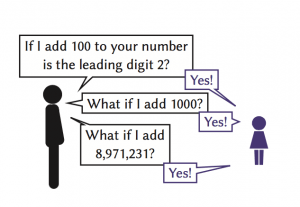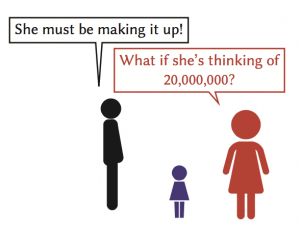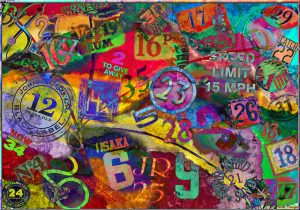# Pretend numbers

20 questions, the axiom of choice and colouring sequences.Image: Adapted from Rust numbers by Andy Maguire, CC-BY 2.0

Some years ago when one of my nieces was quite small, she loved to play a guessing game with the family. She would think of a number (for us that means a whole positive number) and then we we would ask her questions with yes/no answers, trying to guess what her number was. For example, we could ask ‘is it even?’ or ‘is it bigger than 100?’, etc.

Now this game was going on for some time and I was starting to wonder if she actually had a number or was she just pretending (this was by far my cheekiest niece). Pretending to have a number is not too hard when the questions are coming at you one at a time. You just need to make sure you don’t box yourself in. For example, if one of us asked ‘is it bigger than 100?’, then she would have to reply ‘yes’. Otherwise we could just go through all the numbers from 1 to 100 asking ‘is it 1?’, ‘is it 2?’ etc, and when she answered ‘no’ to all of them she would have been caught out.  My niece could definitely pull it off.

## Pretend numbers

A much deeper question is whether she could have decided in advance the answer to every possible yes/no question, without actually having a number.  In order for her to do that her  answers would have to be consistent.  That is, for any possible finite sequence of questions that we might ask, her answers could not contradict themselves.  We will call such a consistent set of answers a pretend number (the technical term is a non-principal ultrafilter).

So do pretend numbers even exist? The answer turns out to be more philosophical than mathematical. A pretend number requires an infinite set of answers to every possible yes/no question. Thus their existence is tied up with the axiom of choice. The axiom of choice is traditionally explained in terms of pairs of identical socks. If I had $3$ such pairs and I wanted to pick one sock from each pair, then I would have $2 \times 2\times2 =8$ ways of doing so.  If I had $5$ pairs of socks then I would have $32$ ways of picking one from each pair. The more pairs of socks I have, the more ways of choosing one from each pair. So it seems reasonable that if I had infinitely many pairs there would certainly be at least one way of choosing one sock from each pair.However, this would require infinitely many choices to be made and it is not clear that this is possible.  Note that the socks are identical, so I cannot for example just pick the larger one from each pair and explain all my choices in one sentence.  The axiom of choice says that infinitely many choices can be made. It cannot be proven or disproven mathematically, so we are free to believe it or not.  Whilst the sock example may make it sound reasonable, it has some pretty unintuitive consequences if you believe it.  For example, the freedom to make infinitely many choices would allow you to cut a solid object into such fuzzy and strange pieces, that you could put them back together and have twice the volume that you started with!

Moreover, if you believe that it is possible to make infinitely many choices then pretend numbers do exist.  In fact, for any consistent set of answers to some yes/no questions there is a pretend number with those properties.

So there is a pretend number $U$, with leading digit 2 such that for any number $n$, if I ask, as shown above, ‘Does $n+U$ have leading digit 2?’, the answer is ‘yes’.Note that no actual number has this property!  If you had selected an actual number, say $2341$, then it would fail as $1000+2341$ does not have leading digit 2.  However these answers are consistent, as for any finite set of values of $n$ that I could ask about, there is always an actual number $m$ that $U$ could be.  That is, $n+m$ all have leading digit 2.  For example, if I say ‘yes, $1000+U$ has leading digit 2, and so does $123456+U$ and $7101202+U$’, then I haven’t contradicted myself as $U$ could be 20,000,000.

On the other hand, if we do not believe in the axiom of choice, then there need be no pretend numbers at all.  The surprising and somewhat controversial thing is that both answers are mathematically correct.  As my undergraduate lecturer put it, if you believe in a mind that can make infinitely many choices then you are more likely to believe in the axiom of choice, whereas if you only believe in the concrete and tangible, you may be more inclined not to.

My niece never told us what her number was.  Did she have a number?  Was she tricking us?  Had she found a way around the philosophical ambiguity of the axiom of choice and come up with a pretend number?  We will never know.

## Properties of pretend numbers

Given the philosophical and even religious ambiguity that surrounds their existence, one can perform surprisingly concrete operations on pretend numbers, such as arithmetic.  If I want to add an actual number $n$ to a pretend number $U$ then I get a pretend number $n+U$.  But what is $n+U$?  Recall that a pretend number is just a collection of consistent answers to every yes/no question about it, so to say what $n+U$ is I just need to be able to answer yes/no questions about it.  But a question about $n+U$ is really just a question about $U$, and we know all the answers to yes/no questions about $U$, since $U$ is a pretend number.  For example, if you ask ‘Is $3+U$ a square number?’, then the answer is just the same as the answer to the question ‘Is $U$ three less than a square?’.Some examples of numbers presented in a very colourful way (Mark Morgan, CC-BY 2.0)

Now for the mind-bending part.  How do I add two pretend numbers? What is $U+V$?  Again I just need to be able to answer yes/no questions about $U+V$. Consider a yes/no question: ‘Does the number have property $A$?’ (eg $A$ could be the property of being prime or being a square).  For any actual number $u$, we have just seen how to answer for $u+V$.  So we can ask ‘Is $U$ one of the actual numbers $u$, such that $u+V$ has property $A$?’  We know the answer, as by definition we know the answer to all yes/no questions about $U$.

So we say that $U+V$ has property $A$ precisely when $U$ has the property of actual numbers $u$ that $u+V$ has property $A$.

This takes a bit of getting your head around, but is not as bad as it seems. Let us look at an example. Suppose Umar is playing the guessing game and is thinking of the number $2$, and Vicki is playing the game and thinking of the number $3$.  Then if we add their numbers via the process just described we would hope to get $5$ (so our notion of addition agrees with addition of actual numbers).  Let’s see if that is indeed the case:

The sum has a property $A$ precisely when Umar’s number has the property of numbers $u$ that $u+V$ has property $A$ (where $V$ denotes Vicki’s number).  As Umar is thinking of the number $2$, he would answer yes precisely if $2+V$ has property $A$.  This is the case precisely when $V$ has the property of actual numbers $v$ that $2+v$ has property $A$.  As Vicki is thinking of $3$, she would answer yes precisely if $2+3$ has property $A$.

In summary, the sum of Umar and Vicki’s numbers has a property precisely when $2+3=5$ has that property.  In particular, if you ask if the sum is $5$, then the answer is yes.  Thus the sum of Umar and Vicki’s numbers is indeed $5$ as we had hoped.

Addition of pretend numbers behaves quite sensibly in many ways.  For pretend numbers $U, V, W$ we have $$(U+V)+W= U+(V+W)$$ as you would expect.  Also for an actual number $n$ we have $n+U=U+n$.  The big shock is that there exist pretend numbers $U$ and $V$ with $$U+V \neq V+U.$$
For example, we have already seen that we can have a pretend number $U$ with leading digit 2, such that for any actual number $n$ the leading digit of $n+U$ is still 2.  Similarly we can have a pretend number $V$ with leading digit 5, such that for any actual number $n$ the leading digit of $n+V$ is still 5. So what is the leading digit of $U+V$?

Once we accept the axiom of choice we are able to assume the existence of pretend numbers…

For any actual number $u$ the leading digit of $u+V$ is 5, so if you ask if $U$ has this property, I would have to say `yes’, or you would have caught me out as not having an actual number.  So the leading digit of $U+V$ is 5.  But by the same logic the leading digit of $V+U$ is 2, so they cannot be the same!

Once we accept the axiom of choice we are able to assume the existence of pretend numbers with desired properties and do calculations with them as above.  However we should bear in mind that we can never actually specify a pretend number.  We cannot specify consistent answers to all the infinitely many yes/no questions one might ask about it.  To actually produce a pretend number would contradict the fact that it is not mathematically wrong to say that there are no pretend numbers.

## Applications

Given this intangibleness, it is natural to assume that pretend numbers are an intellectual curiosity, of no relevance to anything else. In fact nothing could be further from the truth.  They play a prominent role in many fields such as mathematical logic, non-standard analysis, point set topology, topological dynamics, geometric group theory, combinatorics and field extensions and products of algebras.

One especially amazing application is in the field of Ramsey theory.  Ramsey theory considers the painting of structures  (such as the connections in a network or whole numbers) with different colours. In particular, it determines which structures are guaranteed to be found in a part painted a single colour.  The usual idea is that if so little of the structure is painted one colour so that what you are looking for cannot be found in that colour, then enough must be painted in the remaining colours that what you are looking for can be found in one of them.  A simple example is that if you paint $11$ balls each either red or green, then $6$ of them must be the same colour.  You do not know if there are $6$ green balls or $6$ red balls, but if you don’t have one then you must have the other.

In order to appreciate the application of pretend numbers to Ramsey theory, I invite you to solve the following puzzle before reading on:

In the box below the numbers from 1 to 30 have been coloured with different colours.  Can you find three distinct numbers that are all the same colour and all the same colour as any sum of some of them?Hopefully that wasn’t too hard.  Now suppose that the numbers from 1 to 1,000,000 are coloured red, green, blue and yellow.  Could you guarantee that no matter how they are coloured, you can still find three distinct numbers, all the same colour and whose sums are all the same colour?  It is much harder when you do not know how the numbers are coloured, as you must account for every possible colouring.University of California, Berkley (Charlie Nguyen, CC-BY 2.0)

Now suppose that all the numbers, $1,2,3,\ldots,$ are coloured with a finite number of colours.  Instead of finding just three numbers, could you guarantee that there is an infinite set of numbers, so that if you add any finite combination of them together, the result is always the same colour?

In 1970 this was an open problem that combinatorialists were seeking a solution to.  Around this time a young mathematician, Steven Glazer of Berkeley, thought of a short and elegant proof that however all the numbers are coloured, such an infinite set can always be found.  However his proof would only work if there were a pretend number $U$ with $U+U=U$.

Not knowing if a pretend number with the required property existed, Steven Glazer did not publish his work.  In 1974 Neil Hindman finally proved that such an infinite set can always be found, via a much more complicated proof.  The result now bears his name: Hindman’s Theorem.

A year later Steven Glazer mentioned his proof to another mathematician, Fred Galvin.  Now, Fred Galvin knew about compact semigroups.  These structures arose in an area of mathematics known as dynamical systems, which grew out of the study of how physical systems evolve over time-far away from the world of colouring in whole numbers, one might think.

Don’t worry if you don’t know what a non-empty compact semicontinuous semigroup is.  What is important is that the pretend numbers are one (assuming the axiom of choice) and Fred knew it.  Further he knew that in  any  such semigroup, one can solve $U+U=U$.

Note no actual number has this property, as when you add an actual number to itself it gets bigger.  However, using mathematics from this very different field, one can deduce that there is a pretend number with this property. Thus Steven Glazer’s proof was valid.

## Glazer’s proof

Suppose all the positive whole numbers have been coloured with some finite set of colours.  Let $U$ be a pretend number with the property that $U+U=U$.  We can ask if $U$ is red.  If not we can ask if it is green.  Proceeding through all the colours, the answer must at some point be yes (or the answers would not be consistent and the pretend number $U$ would be revealed as fake).

Suppose then that $U$ is blue.  As $U+U=U$, it is not saying anything extra to add that $U+U$ is blue.  Thus $U$ satisfies the property of actual numbers $u$ that ‘$u$ is blue and $u+U$ is blue’.  Thus there must actually be a number $x_1$ with this property (or $U$ is revealed as fake).
Now we know the following are blue:

$U$, $U+U$, $x_1$, $x_1+U$, $x_1+U+U$.

Rearranging we have the following all blue:

$U$, $U+U$, $x_1$, $U+x_1$, $U+ x_1+U$.

Let $FS$ of a sequence be the set of sums of distinct terms.  So we may make the above statement more succinctly by saying that the $FS(x_1,U,U)$ are all blue. So $U$, has the property of actual numbers $u$ that the following are all blue:

$u$, $u+U$, $x_1$, $u+x_1$, $u+x_1+U$.

Again there must actually be such a number $x_2$, or $U$ would be revealed as fake. Thus  $FS(x_1,x_2,U)$ are blue. We proceed in this way by induction.

Suppose we have established that $FS(x_1,\ldots, x_n,U)$ are all blue. As $U=U+U$, we know that  $FS(x_1,\ldots, x_n,U,U)$ are all blue.  Thus there must actually be a number $x_{n+1}$ so that  $$FS(x_1,\ldots, x_n,x_{n+1}) \qquad {\rm and} \qquad FS(x_1,\ldots, x_n,x_{n+1}+U)$$ are all blue.  We already knew that  $FS(x_1,\ldots, x_n,U)$ are all blue, so combining all three sets we get that  $FS(x_1,\ldots, x_n,x_{n+1},U)$ are all blue.

Thus we obtain an infinite sequence $x_1,x_2,x_3,\ldots$, whose sums of distinct terms are all blue.  Either we have a subsequence of infinitely many distinct $x_i$ or some value $x$ gets repeated infinitely often.  In the latter case all finite sums of distinct terms of the sequence $x,2x,3x,4x,\ldots$ are blue.

Either way we have infinitely many distinct numbers, with any sum of distinct numbers being blue and the proof is complete.

## And so…

Steven Glazer found out that his solution works, one year after the problem had been solved by someone else.  Nonetheless his proof is much celebrated by mathematicians for its shortness, its elegance and how it demonstrates the importance of mathematicians sharing ideas from different fields.  In the words of George Bernard Shaw: “If you have an apple and I have an apple and we exchange apples then you and I will still each have one apple. But if you have an idea and I have an idea and we exchange these ideas, then each of us will have two ideas.”Wajid is a lecturer at Queen Mary University of London. He works in low dimensional topology, focusing on the question of when it is possible to completely flatten solid objects.